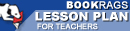Lesson Plans

# The Logic of Scientific Discovery Test | Mid-Book Test - Medium

This set of Lesson Plans consists of approximately 102 pages of tests, essay questions, lessons, and other teaching materials.
 View a FREE sampleName: _________________________ Period: ___________________

This test consists of 5 multiple choice questions, 5 short answer questions, and 10 short essay questions.

## Multiple Choice Questions

1. What can observation do for science?
(a) Assume falsification.
(b) Establish the truth of a statement.
(c) Provide information on facts.
(d) Justify statements.

2. What are the boundaries of conditions?
(a) Theory and proof.
(b) Truth and falsehood.
(c) Logic and non-logic.
(d) Physical and metaphysical.

3. What does the first rule of logic do?
(a) Determines the type of research.
(b) States testability.
(c) Proves a scientific statement.
(d) Sets a norm.

4. Popper rejects perceptions because they tie into what type of principles?
(a) Inductive.
(b) Deductive.
(c) Logical.
(d) Metaphysical.

5. For a theory to be falsifiable, what must it prohibit?
(a) At least one occurrence.
(b) Logic.
(c) Previous theories.
(d) Conventionalism.

## Short Answer Questions

1. What is the theory of theories?

2. What is a precondition for rational consideration?

3. Natural laws are in a form to negate what type of statements?

4. What type of reasoning does Popper deny exists?

5. What does Popper feel is a fruitless exercise?

## Short Essay Questions

1. What is the falsification of a statement?

2. What is Fries' trilemma?

3. Popper states that scientific statements cannot be conclusively disproved. If this is the case what can be determined with these statements?

4. How does one make a statement falsifiable?

5. With regard to universal and singular statements, what does Popper note about symbolic logic?

6. What does Popper classify as being inductive statements?

7. How does metaphysics factor into the scientific process?

8. Why does Popper discredit the conventionalist view of science?

9. Why is the concept of numerical universality a problem for Popper?

10. What argument does Popper present against conventionalists and positivists regarding the testing of a theory?

(see the answer keys)

 This section contains 708 words (approx. 3 pages at 300 words per page) View a FREE sampleMore summaries and resources for teaching or studying The Logic of Scientific Discovery.